# RD Sharma Solutions for Class 6 Maths Chapter 10: Basic Geometrical Concepts Exercise 10.2

The primary objective of preparing exercise-wise solutions is to provide a better learning experience for the students. RD Sharma Solutions Class 6 consists of answers with diagrammatic representation to make it easier for the students for their exam preparation. This exercise has problems based on line segments and their comparison. The students can refer to RD Sharma Solutions for Class 6 Maths Chapter 10 Basic Geometrical Concepts Exercise 10.2 PDF while solving textbook problems.

## RD Sharma Solutions for Class 6 Maths Chapter 10: Basic Geometrical Concepts Exercise 10.2 Download PDF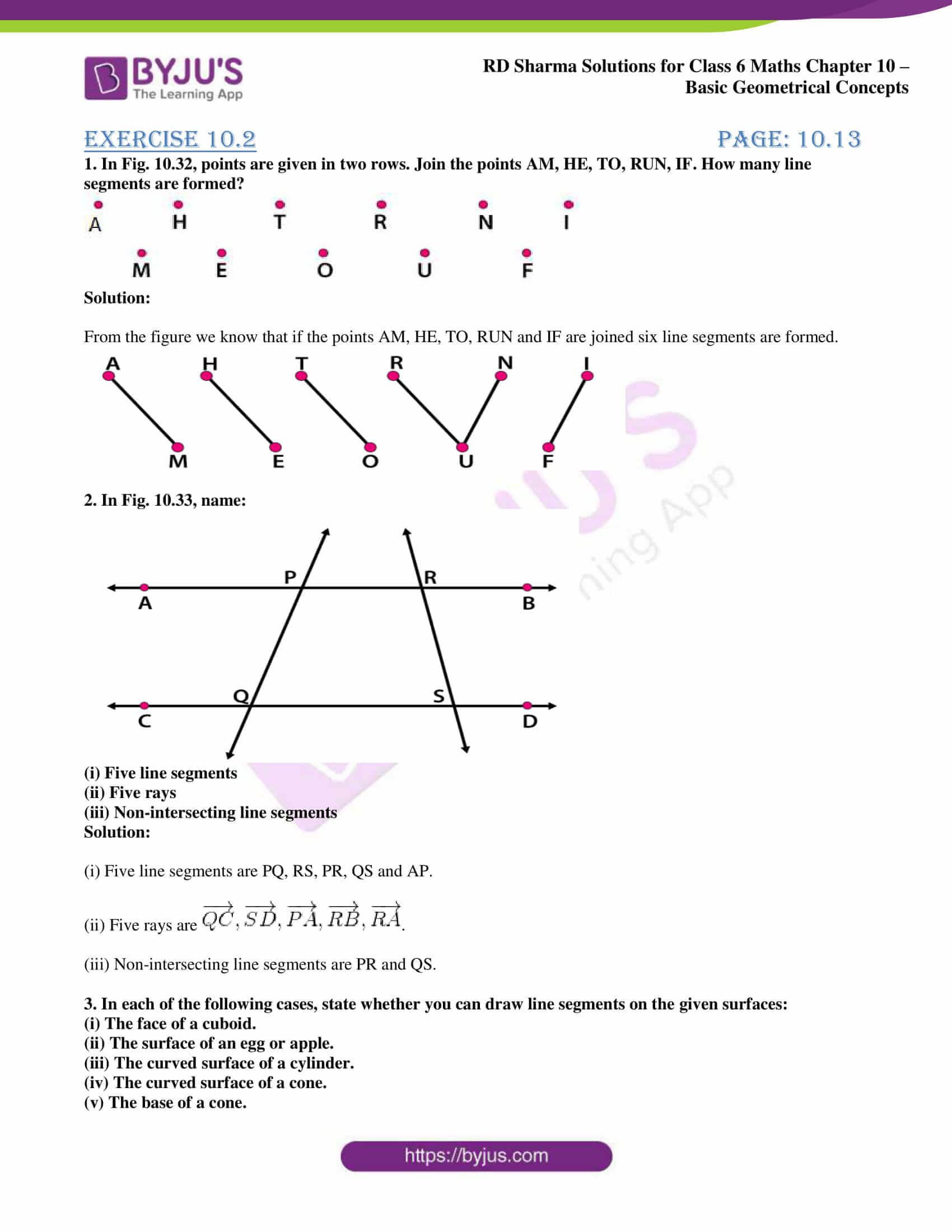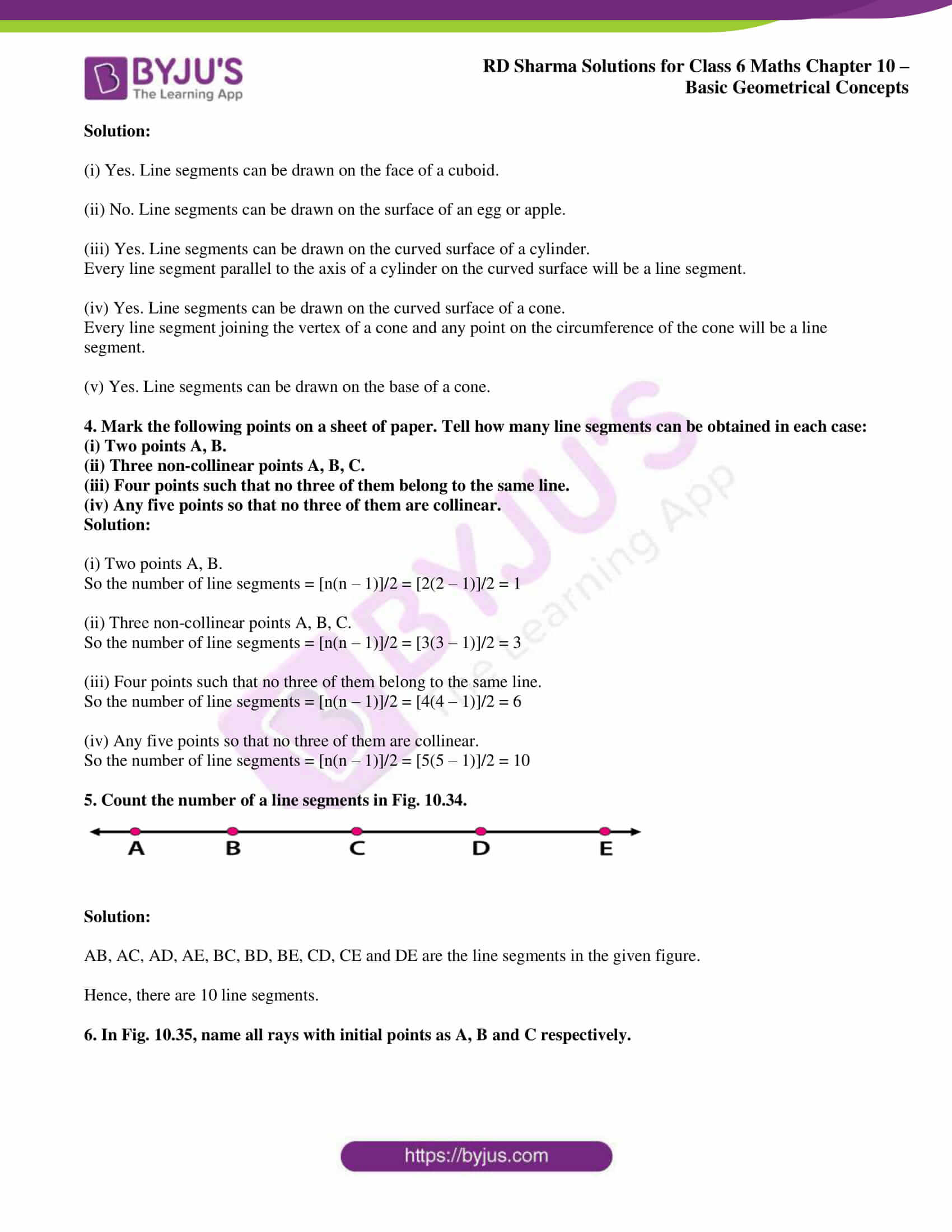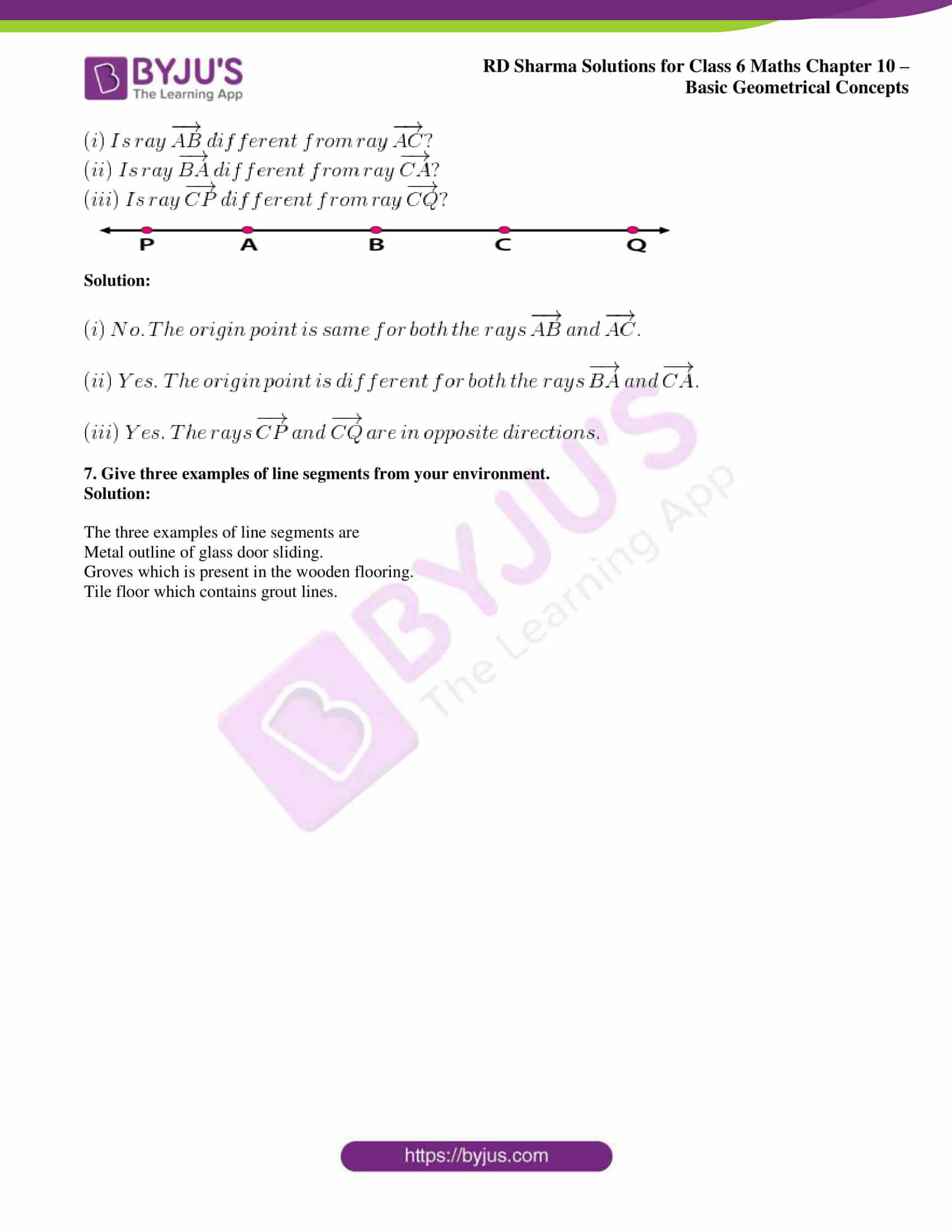### Access answers to Maths RD Sharma Solutions for Class 6 Chapter 10: Basic Geometrical Concepts Exercise 10.2

1. In Fig. 10.32, points are given in two rows. Join the points AM, HE, TO, RUN, IF. How many line segments are formed?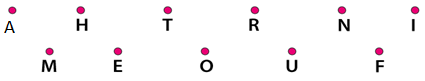Solution:

From the figure we know that if the points AM, HE, TO, RUN and IF are joined six line segments are formed.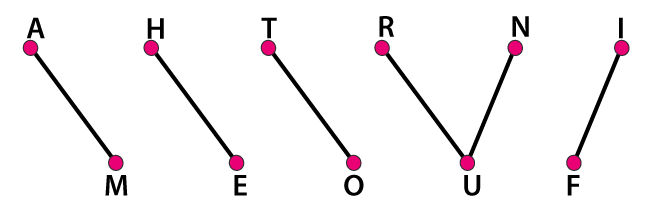2. In Fig. 10.33, name: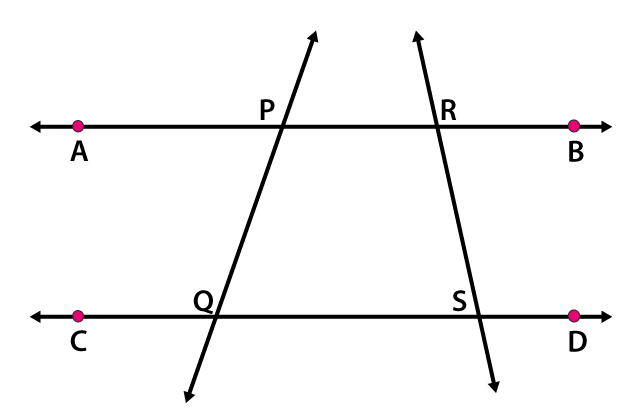(i) Five line segments

(ii) Five rays

(iii) Non-intersecting line segments

Solution:

(i) Five line segments are PQ, RS, PR, QS and AP.

(ii) Five rays are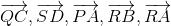.

(iii) Non-intersecting line segments are PR and QS.

3. In each of the following cases, state whether you can draw line segments on the given surfaces:

(i) The face of a cuboid.

(ii) The surface of an egg or apple.

(iii) The curved surface of a cylinder.

(iv) The curved surface of a cone.

(v) The base of a cone.

Solution:

(i) Yes. Line segments can be drawn on the face of a cuboid.

(ii) No. Line segments can be drawn on the surface of an egg or apple.

(iii) Yes. Line segments can be drawn on the curved surface of a cylinder.

Every line segment parallel to the axis of a cylinder on the curved surface will be a line segment.

(iv) Yes. Line segments can be drawn on the curved surface of a cone.

Every line segment joining the vertex of a cone and any point on the circumference of the cone will be a line segment.

(v) Yes. Line segments can be drawn on the base of a cone.

4. Mark the following points on a sheet of paper. Tell how many line segments can be obtained in each case:

(i) Two points A, B.

(ii) Three non-collinear points A, B, C.

(iii) Four points such that no three of them belong to the same line.

(iv) Any five points so that no three of them are collinear.

Solution:

(i) Two points A, B.

So the number of line segments = [n(n – 1)]/2 = [2(2 – 1)]/2 = 1

(ii) Three non-collinear points A, B, C.

So the number of line segments = [n(n – 1)]/2 = [3(3 – 1)]/2 = 3

(iii) Four points such that no three of them belong to the same line.

So the number of line segments = [n(n – 1)]/2 = [4(4 – 1)]/2 = 6

(iv) Any five points so that no three of them are collinear.

So the number of line segments = [n(n – 1)]/2 = [5(5 – 1)]/2 = 10

5. Count the number of a line segments in Fig. 10.34.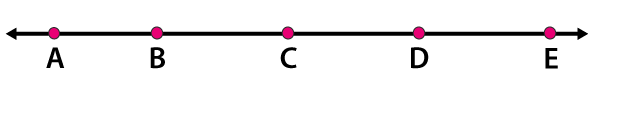Solution:

AB, AC, AD, AE, BC, BD, BE, CD, CE and DE are the line segments in the given figure.

Hence, there are 10 line segments.

6. In Fig. 10.35, name all rays with initial points as A, B and C respectively.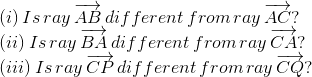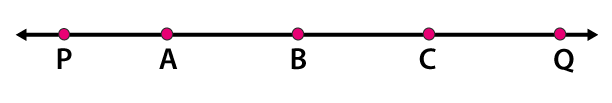Solution: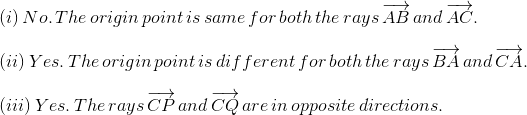7. Give three examples of line segments from your environment.

Solution:

The three examples of line segments are

Metal outline of glass door sliding.

Groves which is present in the wooden flooring.

Tile floor which contains grout lines.